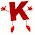# GATE Questions-DBMS-ER Diagram

Previous GATE questions with solutions on DBMS (ER Diagram) - CS/IT

GATE-2008
1. Consider the following ER diagram.
The minimum number of tables needed to represent M, N, P, R1, R2 is
(a) 2  (b) 3  (c) 4  (d) 5

Ans: confused between option (a) & option (b)   { majority of the people refer option (a) as correct }
Explanation:
for option(a)
check explanation of question (2) of this link http://www.geeksforgeeks.org/database-management-systems-set-7/

for option (b)
All strong entities and weak entities will be converted into a table. Therefore we will have 3 tables:
M (M1,M2,M3,P1)
P (P1,P2)
N (N1,N2,P1) =>N is a weak entity and it is modified to include the primary key of P (i.e. P1).

2. Which of the following is a correct attribute set for one of the tables for the correct answer to the above question?
(a) {M1, M2, M3, P1}
(b) {M1, P1, N1, N2}
(c) {M1, P1, N1}
(d) {M1, P1}

Ans: option (a)

GATE-2005
3. The following table has two attributes A and C where A is the primary key and C is the foreign key referencing A with on-delete cascade.
-----
A   C
-----
2   4
3   4
4   3
5   2
7   2
9   5
6   4
-----
The set of all tuples that must be additionally deleted to preserve referential integrity when the tuple (2,4) is deleted is:
(a) (3,4) and (6,4)
(b) (5,2) and (7,2)
(c) (5,2), (7,2) and (9,5)
(d) (3,4), (4,3) and (6,4)

Ans: option (c)
Explanation:
Note that C is a foreign key, referring A with delete on cascade. Therefore when (2,4) is deleted, all the rows with value 2 in field C also should be deleted. Hence (5,2) and (7,2) is also deleted. Now rows with value 5 and 7 in field C also should be deleted. Therefore (9,5) is also deleted.

GATE-2005
4. Let E1 and E2 be two entities in an ER diagram with simple single-valued attributes. R1 and R2 are two relationships between E1 and E2, where R1 is one-to-many and R2 is many-to-many. R1 and R2 do not have any attributes of their own. What is the minimum number of tables required to represent this situation in the relational model?
(a) 2    (b) 3    (c) 4    (d) 5

Ans: option (b)
Explanation:
Strong entities E1 and E2 should be converted into tables. For R1, which is one to many relation, there is no need of a separate table. Modify the "many" side to include the primary key of "one" side as foreign key. For R2, which is many to many relation, we require a separate table by including the primary key of E1 and E2 as foreign keys. Hence we require a minimum of 3 tables.

GATE-2012
5.  Given the basic ER and relational models, which of the following is INCORRECT?
(a) An attribute of an entity can have more than one value
(b) An attribute of an entity can be composite
(c) In a row of a relational table, an attribute can have more than one value
(d) In a row of a relational table, an attribute can have exactly one value or a NULL value

Ans: option (c)
Explanation:
According to First Normal Form, an attribute cannot have multiple values.

GATE-2011
6. Consider a relational table with a single record for each registered student with the following attributes.
1. Registration_Number: Unique registration number of each registered student
2. UID: Unique Identity number, unique at the national level for each citizen
3. BankAccount_Number: Unique account number at the bank. A student can have multiple accounts or joint accounts. This attributes stores the primary account number
4. Name: Name of the Student
5. Hostel_Room: Room number of the hostel
Which of the following options is INCORRECT?
(a) BankAccount_Number is a candidate key
(b) Registration_Number can be a primary key
(c) UID is a candidate key if all students are from the same country
(d) If S is a superkey such that S UID is NULL then S U UID is also a superkey

Ans: option (a)
Explanation:
Candidate Key: All unique value columns in a table are called candidate keys.
Its already specified in the question that "A student can have multiple accounts or joint accounts". Hence if two students have a joint account, BankAccount_Number will be the same both the students. Hence BankAccount_Number cannot be a candidate key.

GATE-2015
7. Consider an Entity-Relationship (ER) model in which entity sets E1 and E2 are connected by an m : n relationship R12, E1 and E3 are connected by a 1 : n (1 on the side of E1 and n on the side of E3) relationship R13.
E1 has two single-valued attributes a11 and a12 of which a11 is the key attribute. E2 has two single-valued attributes a21 and a22 of which a21is the key attribute. E3 has two single-valued attributes a31 and a32 of which a31 is the key attribute. The relationships do not have any attributes.
If a relational model is derived from the above ER model, then the minimum number of relations that would be generated if all the relations are in 3NF is ___________.

Ans: 4
Explanation:
Entities  E1, E2 and E3 each requires one table.
Now relation R12 is m:n .Hence requires a table. R(a11, a21)
R13 is 1:n.
To map 1:n relationships, the primary key on the 'one side' of the relationship is added to the 'many side' as a foreign key. (reference: http://db.grussell.org/section006.html)
Hence table for E3 is modified to include a11 as a foreign key.
Hence
E1(a11, a12)
E2(a21, a22)
E3(a31, a32, a11)
R13(a11, a13)
The relation is still in 3NF as for  X -> A, if one of the following holds
1) X is a superkey or
2) A-X is a prime attribute

Question 7 contributed by Radhika V.M., Mtech, College of Engineering Trivandrum

1.2.